Question

# A long wire carrying a 6.00A current reverses direction by means of two right-angle bends, as...

A long wire carrying a 6.00A current reverses direction by means of two right-angle bends, as shown in the figure (Figure 1) . The part of the wire where the bend occurs is in a magnetic field of 0.666T confined to the circular region of diameter 75cm, as shown.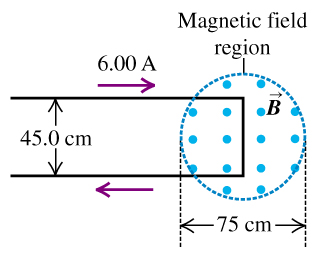A) Find the direction of the net force that the magnetic field exerts on this wire.

B) Find the magnitude of the net force that the magnetic field exerts on this wire.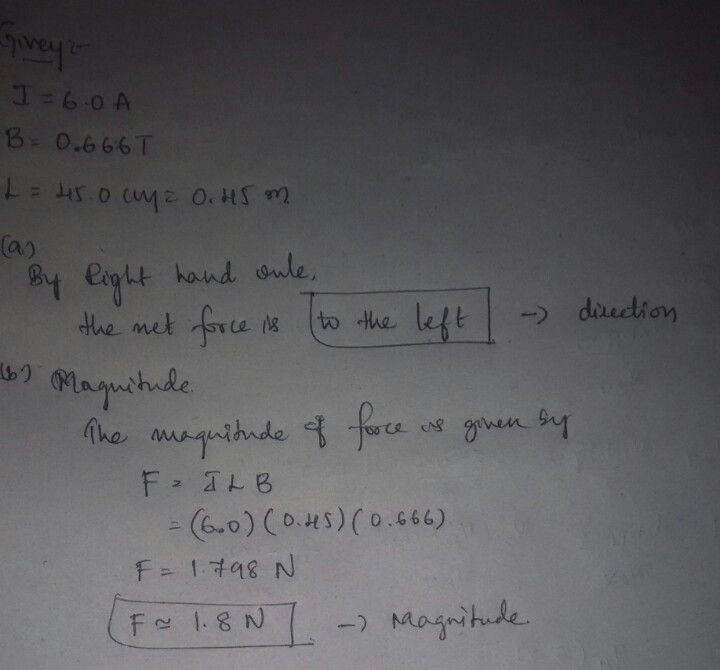#### Earn Coins

Coins can be redeemed for fabulous gifts.

Similar Homework Help Questions
• ### A long wire carrying 6.50 A of current makes two bends, as shown in the figure.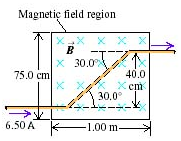A long wire carrying 6.50 A of current makes two bends, as shown in the figure. The bent part of the wire passes through a uniform 0.280 T magnetic field directed as shown in the figure and confined to a limited region of space.A) Find the magnitude of the force that the magnetic field exerts on the wire.B) Find the direction of the force that the magnetic field exerts on the wire.

• ### ​A long wire carrying 6.50 A of currents makes two bends, as shown in the figure on the right.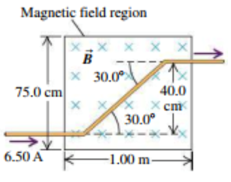A long wire carrying 6.50 A of currents makes two bends, as shown in the figure on the right. The bent part of the wire passes through a uniform 0.280-T magnetic field directed as shown and confined to a limited region of space. a. What are the magnitude and direction of the force exerted on the wire by the magnetic field?b. Now assume that the magnetic field extends 1.00 m to the left and to the right of the region shown...

• ### A long wire carrying 4.50 A of current makes two 90 degree bends, as shown in....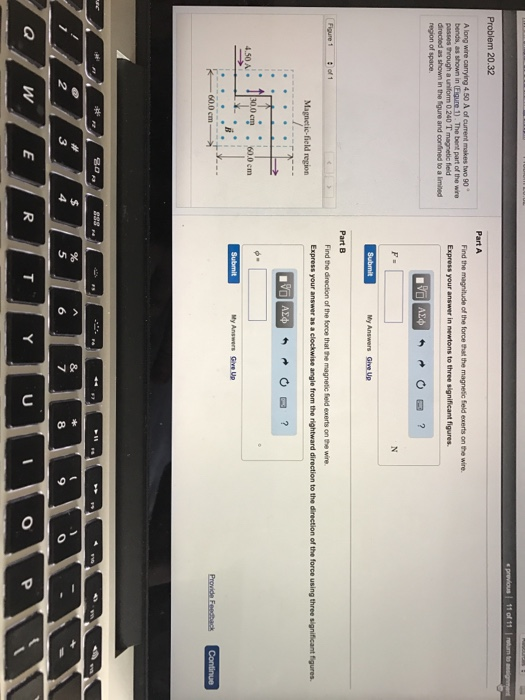A long wire carrying 4.50 A of current makes two 90 degree bends, as shown in. The best part of the wire passes through a uniform 0.240 t magnetic field directed as shown in the figure and confined to a limited of space. Part A Find the magnitude of the force that the magnetic field exerts on the wire Part B Find the direction of the force that the magnetic field exerts on the wire.

• ### A long wire carrying 4.50 A of current makes two 90 bends, as shown in the...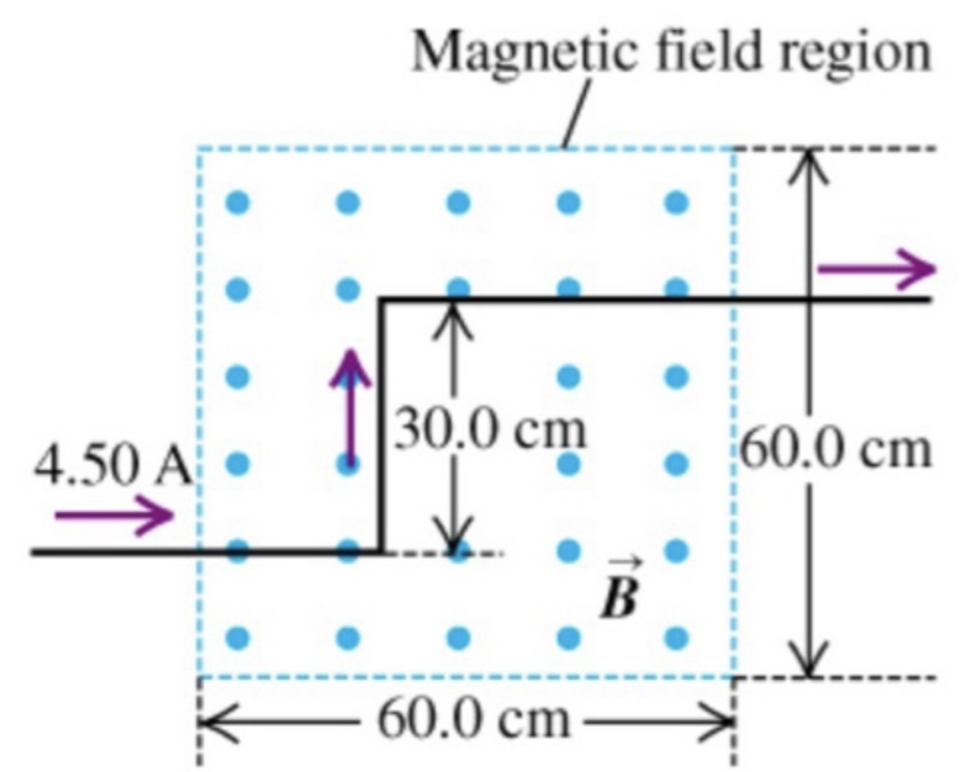A long wire carrying 4.50 A of current makes two 90° bends, as shown in the figureThe bent part of the wire passes through a uniform 0.238 T magnetic field directed as shown in the figure and confined to a limited region of space.Part AFind the magnitude of the force that the magnetic field experts on the the wire.Part BFind the direction of the force that the magnetic field experts on the the wire.

• ### A wire carrying a 31.0 A current bends through a right angle.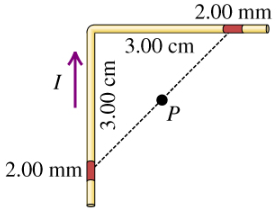Exercise 28.13 - Enhanced - with Solution A wire carrying a 31.0 A current bends through a right angle. Consider two 2.00 mm segments of wire, each 3.00 cm from the bend (Figure 1). Part A Find the magnitude of the magnetic field these two segments produce at point P, which is midway between them. Express your answer with the appropriate units. Part B Find the direction of the magnetic field at point P. out of the page into the page

• ### Problem 4 Magnetic field region Fig. 2 A long wire carrying 4.50 A of current makes...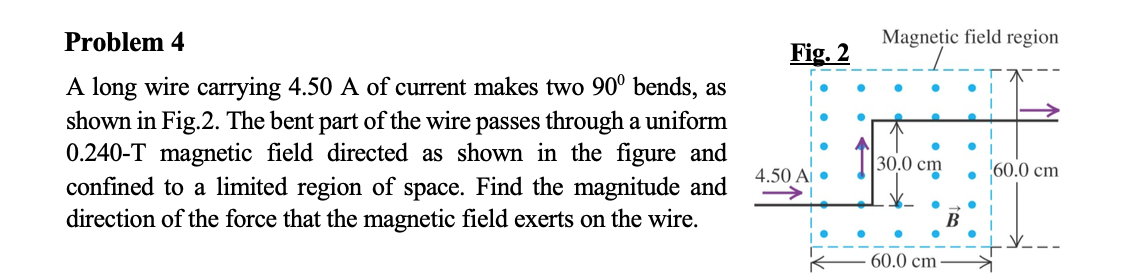Problem 4 Magnetic field region Fig. 2 A long wire carrying 4.50 A of current makes two 90° bends, as shown in Fig.2. The bent part of the wire passes through a uniform 0.240-T magnetic field directed as shown in the figure and confined to a limited region of space. Find the magnitude and direction of the force that the magnetic field exerts on the wire. . ||30.0 cm 60.0 cm 4.5 Y_ - 60.0 cm

• ### There is a straight wire 0.2 m long carrying current 20 mA at angle 30^degree to...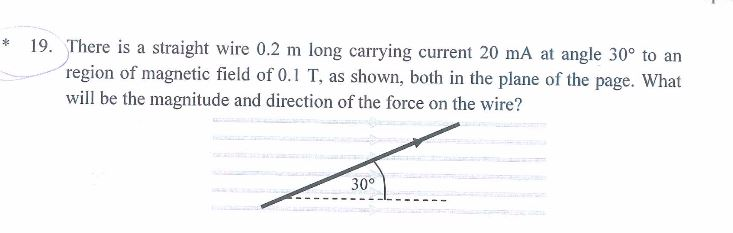There is a straight wire 0.2 m long carrying current 20 mA at angle 30^degree to an region of magnetic field of 0.1 T, as shown, both in the plane of the page. What will be the magnitude and direction of the force on the wire?

• ### A uniform magnetic field of magnitude 25 points to the right. A 2.0-m-long wire, carrying 20...A uniform magnetic field of magnitude 25 points to the right. A 2.0-m-long wire, carrying 20 A, is placed at an angle of to the magnetic field, as shown in the figure. 30° What is the direction of the magnetic force on the wire? The magnetic force points O A to the right; OB out of the page: Cinto the page: OD to the left; E upward; OF downward

• ### 4. A wire carrying a 27.0 A current bend 2.00 mm segments of wire, each 3.00...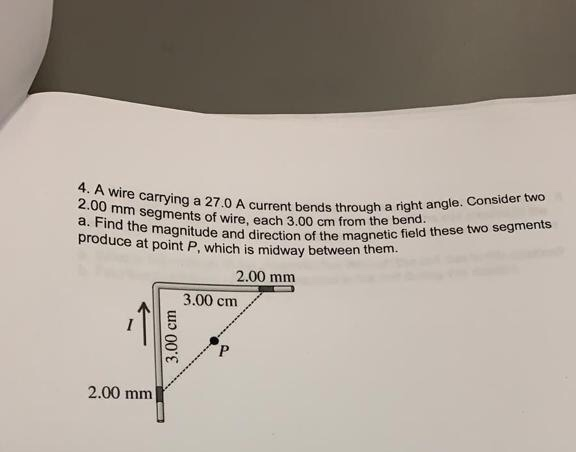4. A wire carrying a 27.0 A current bend 2.00 mm segments of wire, each 3.00 cm from a. Find the magnitude and direction produce at point P, which is midway between them rent bends through a right angle. Consider two re, each 3.00 cm from the bend. and direction of the magnetic field these two segments 2.00 mm 3.00 cm 3.00 cm 2.00 mm